• 写于09年08月29日，函数功能，用MATLAB实现的FFT函数。完整程序function xn=myfft(xn,N,M)mybitrevorder(xn,N);for m=1:MB=2^(m-1);for r=0:B-1P=2^(M-m)*r;for k=r:2^m :N-1f= xn(k+1);xn(k+1)= xn(k+1)+ xn(k+B+1)...

写于09年08月29日，函数功能，用MATLAB实现的FFT函数。
完整程序
function xn=myfft(xn,N,M)
mybitrevorder(xn,N);
for m=1:M
B=2^(m-1);
for r=0:B-1
P=2^(M-m)*r;
for k=r:2^m :N-1
f= xn(k+1);
xn(k+1)= xn(k+1)+ xn(k+B+1)*exp(-i*pi*P/N);
xn(k+B+1)= f-xn (k+B+1)*exp(-i*pi*P/N);
end
end
end
倒序
function xn=mybitrevorder(xn,N)
LH=N/2;
r=LH;
N1=N-2;
for t=1:N1
if t
T=xn(t+1);
xn(t+1)=xn(r+1);
xn(r+1)=T;
end
K=LH;
while r>=K
r=r-K;
K=K./2;
end
r=r+K;
end
综合起来是：
fft程序
function xn=myfft(xn,N,M)
LH=N/2;
r=LH;
N1=N-2;
for t=1:N1
if t
T=xn(t+1);
xn(t+1)=xn(r+1);
xn(r+1)=T;
end
K=LH;
while r>=K
r=r-K;
K=K./2;
end
r=r+K;
end
for m=1:M
B=2^(m-1);
for r=0:B-1
P=2^(M-m)*r;
for k=r:2^m :N-1
f= xn(k+1);
xn(k+1)= xn(k+1)+ xn(k+B+1)*exp(-i*pi*P/N);
xn(k+B+1)= f-xn(k+B+1)*exp(-i*pi*P/N);
end
end
end

展开全文• 信号采集与处理--MATLAB窗函数及其特征讲述PAGE \* MERGEFORMAT11信号采集与处理MATLAB 窗函数及其特征数字信号处理中通常是取其有限的时间片段进行分析，而不是对无限长的信号进行测量和运算。具体做法是从信号中...

信号采集与处理--MATLAB窗函数及其特征讲述
PAGE \* MERGEFORMAT11
信号采集与处理
MATLAB 窗函数及其特征数字信号处理中通常是取其有限的时间片段进行分析，而不是对无限长的信号进行测量和运算。具体做法是从信号中截取一个时间片段，然后对信号进行傅里叶变换、相关分析等数学处理。信号的截断产生了能量泄漏，而用FFT算法计算频谱又产生了栅栏效应，从原理上讲这两种误差都是不能消除的。在FFT分析中为了减少或消除频谱能量泄漏及栅栏效应，可采用不同的截取函数对信号进行截短，截短函数称为窗函数，简称为窗。
泄漏与窗函数频谱的两侧旁瓣有关，对于窗函数的选用总的原则是，要从保持最大信息和消除旁瓣的综合效果出发来考虑问题，尽可能使窗函数频谱中的主瓣宽度应尽量窄，以获得较陡的过渡带；旁瓣衰减应尽量大，以提高阻带的衰减，但通常都不能同时满足这两个要求。频谱中的如果两侧瓣的高度趋于零，而使能量相对集中在主瓣，就可以较为接近于真实的频谱。不同的窗函数对信号频谱的影响是不一样的，这主要是因为不同的窗函数，产生泄漏的大小不一样，频率分辨能力也不一样。信号的加窗处理，重要的问题是在于根据信号的性质和研究目的来选用窗函数。图1是几种常用的窗函数的时域和频域波形，其中矩形窗主瓣窄，旁瓣大，频率识别精度最高，幅值识别精度最低，如果仅要求精确读出主瓣频率，而不考虑幅值精度，则可选用矩形窗，例如测量物体的自振频率等；布莱克曼窗主瓣宽，旁瓣小，频率识别精度最低，但幅值识别精度最高；如果分析窄带信号，且有较强的干扰噪声，则应选用旁瓣幅度小的窗函数，如汉宁窗、三角窗等；对于随时间按指数衰减的函数，可采用指数窗来提高信噪比。表1 是几种常用的窗函数的比较。
如果被测信号是随机或者未知的，或者是一般使用者对窗函数不大了解，要求也不是特别高时，可以选择汉宁窗，因为它的泄漏、波动都较小，并且选择性也较高。但在用于校准时选用平顶窗较好，因为它的通带波动非常小，幅度误差也较小。
5.3 广义余弦窗 汉宁窗、海明窗和布莱克曼窗，都可以用一种通用的形式表示，这就是广义余弦窗。这些窗???是广义余弦窗的特例，汉宁窗又被称为余弦平方窗或升余弦窗，海明窗又被称为改进的升余弦窗，而布莱克曼窗又被称为二阶升余弦窗。采用这些窗可以有效地降低旁瓣的高度，但是同时会增加主瓣的宽度。 这些窗都是频率为0、2π/(N–1)和4π/(N–1)的余弦曲线的合成，其中N为窗的长度。通常采用下面的命令来生成这些窗：Ind=(0:N-1)*2*pi/(N-1)Window=A-B*cos(ind)+C*cos(2*ind)其中，A、B、C适用于自己定义的常数。根据它们取值的不同，可以形成不同的窗函数，分别是： ● 汉宁窗 A=0.5，B=0.5，C=0； ● 海明窗 A=0.54，B=0.54，C=0； ● 布莱克曼窗 A=0.5，B=0.5，C=0.08；
表1 窗函数及其特点与应用名称特点应用bartlett(1) 巴特利特窗是两个矩形窗的卷积。 (2) 此函数生成的巴特利特窗的两端总是0。 (3) 该函数与三角窗函数非常类似。当n是奇数时，则有bartlett(n+2)的中间部分等于triang(n)；当n是偶数时，巴特利特窗的傅立叶变换是负数。布莱克曼窗Blackman二阶升余弦窗，主瓣宽，旁瓣比较低，但等效噪声带宽比汉宁窗要大一点，波动却小一点。频率识别精度最低，但幅值识别精度最高，有更好的选择性。其主瓣宽度是矩形窗主瓣宽度的3倍，为12*pi/N，但其最大旁瓣值比主瓣值低57dB.常用来检测两个频率相近幅度不同的信号。切比雪夫窗(Chebyshev)在给定旁瓣高度下，Chebyshev窗的主瓣宽度最小，具有等波动性，也就是说，其所有的旁瓣都具有相等的高度。切比雪夫窗在边沿的采样点有尖峰。其主瓣值比旁瓣值高r dB.?平顶窗Flap Top平顶窗在频域时的表现就象它的名称一样有非常小的通带波动。由于在幅度上有较小的误差，所以这个窗可以用在校准上。高斯窗Gaussian是一种指数窗。主瓣较宽，故而频率分辨力低；无负的旁瓣，第一旁瓣衰减达一55dB。常被用来截短一些非周期信号，如指数衰减信号等。对于随时间按指数衰减的函数，可采用指数窗来提高信噪比。海明窗(汉明窗)Hamming与汉宁窗都是余弦窗，又称改进的升余弦窗，其主瓣宽度与汉宁窗相同，只是加权系数不同，使旁瓣达到更小。但其旁瓣衰减速度比汉宁窗衰减速度慢。海明窗函数的最大旁瓣值比主瓣值低41dB，但它和汉宁窗函数的主瓣宽度是一样大的。与汉明窗类似，也是很有用的窗函数。汉宁窗Hanning又称升余弦窗。主瓣加宽并降低，旁瓣则显著减小，从减小泄漏观点出发，汉宁窗优于矩形窗．但汉宁窗

展开全文• ## FFT中常用窗函数

万次阅读 2019-06-12 11:16:46
1. 窗函数简介 如果连续时间信号 Xa(t) 在时域无限长，则离散化后的序列 X(n) 也是无限长的，而 DFT 只适用于有限长序列的计算，因此需要对 X(n) 加窗截断，使之成为有限长序列 XN(n)，这个过程称为时域加窗（time-...
1. 窗函数简介
如果连续时间信号 Xa(t) 在时域无限长，则离散化后的序列 X(n) 也是无限长的，而 DFT 只适用于有限长序列的计算，因此需要对 X(n) 加窗截断，使之成为有限长序列 XN(n)，这个过程称为时域加窗（time-windowing）。设窗函数为Wn(N)，则
XN(n) = X(n) * WN(N)
有DFT的性质，时域上有两个序列相乘，在频域上是两个序列的离散时间傅里叶变换的卷积。加窗函数能有效解决傅里叶变换过程中出现的频谱泄露和混叠现象，一般都希望窗函数具有如下两点要求：
（1）主瓣尽可能地窄，以获得较陡的过渡带；
（2）最大旁瓣相对于主瓣尽可能的小，即能尽量集中在主瓣中。
2. 常见窗函数
常见的窗函数：矩形窗（Rectangular window）；汉宁窗（Hanning window）；汉明窗（Hamming window）；布莱克曼窗（Blackman window）。为了定量分析比较各个窗函数的性能，给出这几个窗函数的比较参数。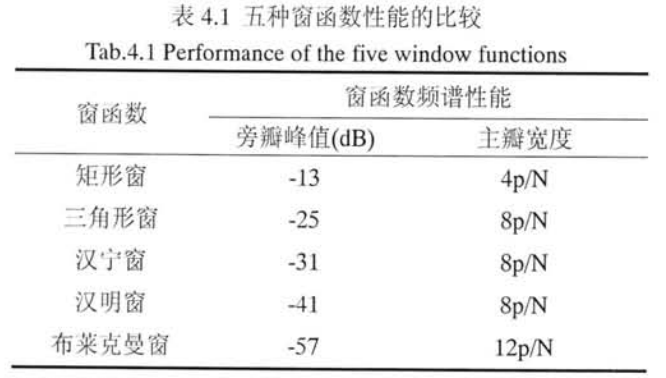对加窗后的信号序列进行FFT时，由于加窗是对信号的不等加权，导致分析结果变换各次谐波幅值将会出现偏差。不同的窗函数的幅值恢复系数，见表4.2：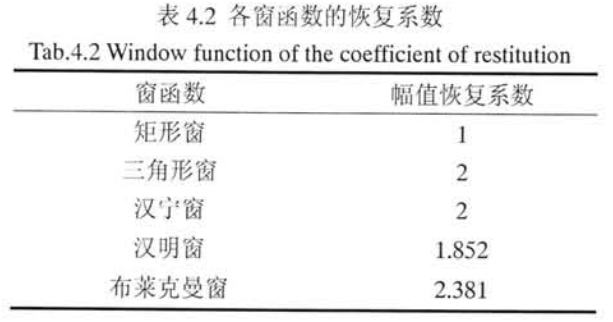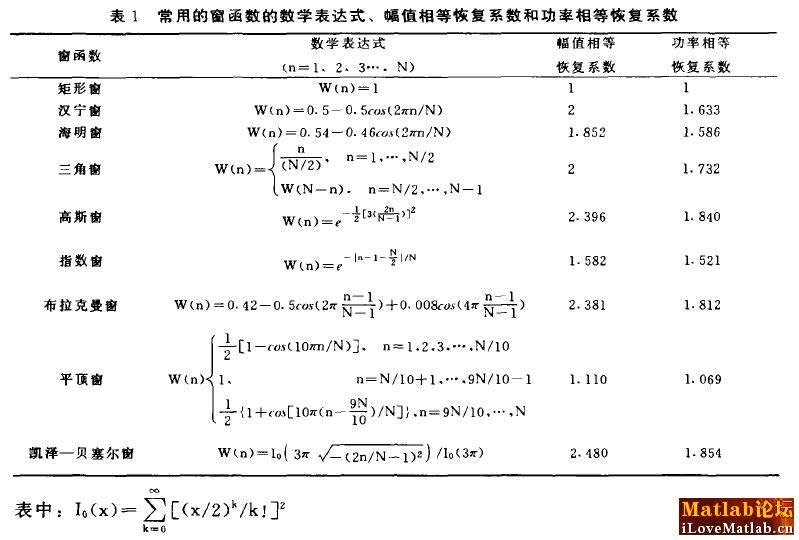展开全文• 窗函数是频谱分析中一个重要的部分，窗函数修正了由于信号的非周期性并减小了频谱中由于泄露而带来的测量不准确性。快速傅里叶变换假定了时间信号是周期无限的。但在分析时，我们往往只截取其中的一部分，因此需要...

窗函数是频谱分析中一个重要的部分，窗函数修正了由于信号的非周期性并减小了频谱中由于泄露而带来的测量不准确性。
快速傅里叶变换假定了时间信号是周期无限的。但在分析时，我们往往只截取其中的一部分，因此需要加窗以减小泄露。窗函数可以加在时域，也可以加在频域上，但在时域上加窗更为普遍。截断效应带来了泄漏，窗函数是为了减小这个截断效应，其设计成一组加权系数。例如，一个窗函数可以定义为：
w(t)=g(t)       -T/2w(t)=0          其他
g(t)是窗函数，T是窗函数的时间.
待分析的数据x(t)则表示为：
x(t)=w(t)*x(t)'
x(t)'表示原始信号,x(t)表示待分析信号。
加窗在时域上表现的是点乘，因此在频域上则表现为卷积。卷积可以被看成是一个平滑的过程。这个平滑过程可以被看出是由一组具有特定函数形状的滤波器，因此，原始信号中在某一频率点上的能量会结合滤波器的形状表现出来，从而减小泄漏。基于这个原理，人们通常在时域上直接加窗。
大多数的信号分析仪一般使用矩形窗(rectangular)，汉宁(hann)，flattop和其他的一些窗函数。
矩形窗函数：
w(k)=1
汉宁窗：
w(k)=0.5*(1-cos(2*pi*k/(N-1)))     0<=k<=N-1
由于加窗计算中衰减了原始信号的部分能量，因此对于最后的结果还需要加上修正系数。在线性谱分析中，一般使用幅度系数(amplitude correction)，在功率谱中，一般使用能量系数(energycorrection)。(这段不清楚在实际中如何用)
matlab中提供了很多窗函数，如下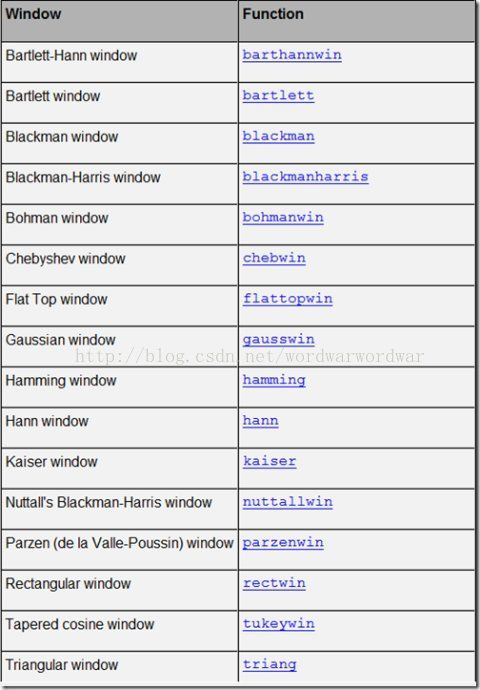还提供了显示窗函数的GUI工具，如wvtool可以显示用来显示窗的形状和频域图形，wintool可以打开窗设计和分析工具，如运行
wvtool(hamming(64),hann(64),gausswin(64))
可以对比汉明窗、汉宁窗和高斯窗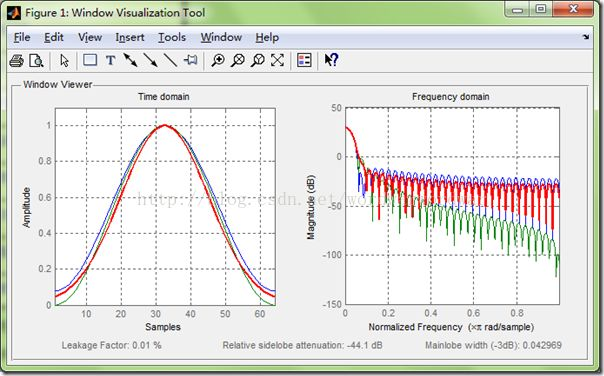简单测试一下加窗的效果如下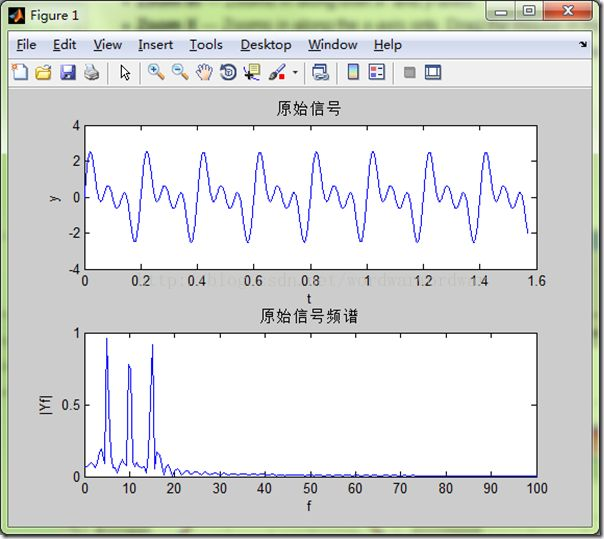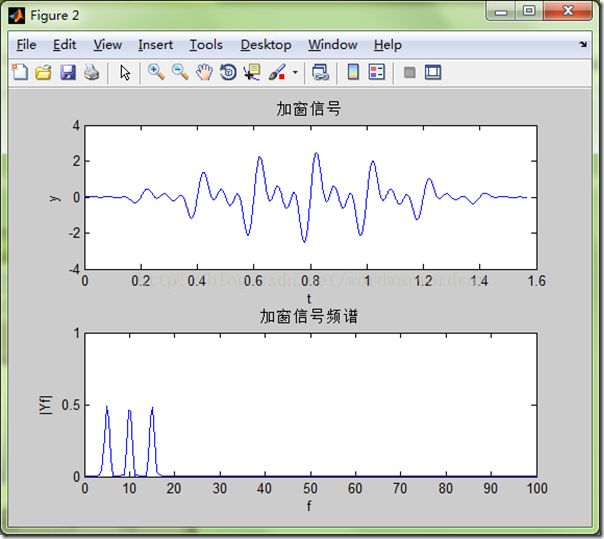可以看到加窗后，频谱泄露确实减少了，但同时信号能量也减小了，这也许就是所说的要使用能量系数吧，如下，这样一来，对比就更明显了，加窗可以有效的减少频谱泄露。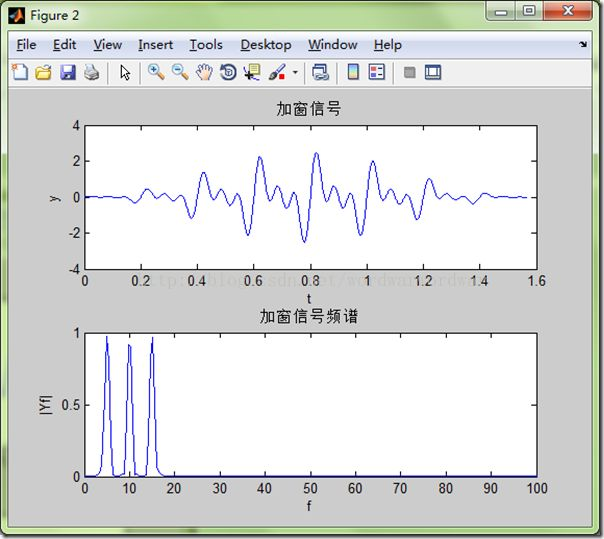测试代码如下
%% 窗函数测试
function main
clc
close all
Ts = 0.001;
Fs = 1/Ts;
%% 原始信号
t = 0:Ts:pi/2;
yt = sin(2*pi*5*t) + sin(2*pi*10*t) + sin(2*pi*15*t);
[Yf, f] = Spectrum_Calc(yt, Fs);
figure
subplot(211)
plot(t, yt)
xlabel('t')
ylabel('y')
title('原始信号')
subplot(212)
plot(f, Yf)
xlabel('f')
ylabel('|Yf|')
xlim([0 100])
ylim([0 1])
title('原始信号频谱')
%% 加窗信号
win = hann(length(t));
yt1 = yt.*win';
[Yf1, f1] = Spectrum_Calc(yt1, Fs);
figure
subplot(211)
plot(t, yt1)
xlabel('t')
ylabel('y')
title('加窗信号')
subplot(212)
plot(f1, 2*Yf1) % 2表示能量系数
xlabel('f')
ylabel('|Yf|')
xlim([0 100])
ylim([0 1])
title('加窗信号频谱')
end
%% 求取频谱
function [Yf, f] = Spectrum_Calc(yt, Fs)
L = length(yt);
NFFT = 2^nextpow2(L);
Yf = fft(yt,NFFT)/L;
Yf = 2*abs(Yf(1:NFFT/2+1));
f = Fs/2*linspace(0,1,NFFT/2+1);
end

展开全文• 采用窗函数法设计理想低通，高通滤波器，参考北京交通大学陈后金主编的【数字信号处理】5.2节 窗函数法设计线性相位FIR数字滤波器P164，和P188。设计步骤如下：1) 确定滤波器类型，不同的FIR类型可设计不同类型的...信号处理
• 定义：﻿MATLAB帮助文件原文The 'i' in the 'Nth root of unity' 是虚数单位​调用：​​1. Y =fft(y);2. Y = fft(y，N);式中，y是序列，Y是序列的快速傅里叶变换。... 函数fft返回值的数据结构具有对...
• ## FFTmatlab 算法 实现

千次阅读 2019-05-09 18:12:10
function [ ret_val ] = FFT1024( vector ) %UNTITLED8 此处显示有关此函数的摘要 % 此处显示详细说明 %UNTITLED7 此处显示有关此函数的摘要 % 此处显示详细说明 %====================================== %ret_val ...
• ## matlab中窗函数的使用(一)

万次阅读 多人点赞 2017-04-02 11:26:46
窗函数是频谱分析中一个重要的部分，窗函数修正了由于信号的非周期性并减小了频谱中由于泄露而带来的测量不准确性。 快速傅里叶变换假定了时间信号是周期无限的。但在分析时，我们往往只截取其中的一部分，因此需要...
• ## 关于矩形窗函数DFT和FFT的笔记

万次阅读 多人点赞 2018-10-28 17:03:19
矩形窗函数的FT是个什么样子呢？ 1、先从理论上分析一下，如图所示（贼简单）： 我们在二维坐标系里只能画出信号的幅度谱 |F(w)| ，那么矩形窗的幅度谱是啥形状呢？（本科的时候快把Sa函数的图画烂了）： 这时，...
• Q1：如何查看matlab fft函数源代码n要取最接近数据长度的2的整数次方，命令是2^nextpow2(n),其中n是实际数据长度，因为这样的n可以使fft更快。比如，n=1021，执行n=2^nextpow2(n)后，n=2^10=1024。而你说的512也是这...
• FFT之前又会用到窗函数。那么各种窗函数之间有什么区别呢？结论放在前面。 窗函数有两个评价指标，主瓣和旁瓣。 如果分析对象是单一频率信号的幅度分辨率比频域分辨率更高，则需要宽的主瓣。 如果分析对象是单一...数字信号处理
• 一，写在前面的话. 学习了以下链接，然后写出了MATLAB代码，在本文末，大家可以随便下载代码，进行尝试，我...自学什么是窗函数，什么是滤波器，为啥要用窗函数，怎么计算一些值，怎么根据这些值选择窗函数。 2. ...FIR滤波器设计
• 几种常见窗函数及其matlab程序实现.doc 几种常见窗函数及其MATLAB程序实现2013121613582296人阅读评论0收藏举报分类MATLAB(15)数字信号处理中通常是取其有限的时间片段进行分析，而不是对无限长的信号进行测量和运算...
• 这里主要是对窗函数的简单应用做些介绍，是在已知滤波器阶数的情况下，设计滤波器。多数情况下，在不能直接知道滤波器阶数的时候，可参考“http://www.cnblogs.com/sunev/archive/2011/11/23/2260579.html”。
• 使用窗函数来截取一段无限长（非常长：...使用窗函数可以减少截断时的噪声（一刀切边缘会产生很多的高频谐波（吉布斯效应），从而造成频谱泄露（FFT的时候会搞出来很多原本信号没有的频率），所以切下去的时候要温柔...信号处理
• ## 几种常见窗函数及其MATLAB实现

万次阅读 多人点赞 2017-03-30 09:34:41
感谢博主 数字信号处理中通常是取其有限的时间片段进行分析，而不是对无限长的信号进行测量和运算。具体做法是从信号中截取一...信号的截断产生了能量泄漏，而用FFT算法计算频谱又产生了栅栏效应，从原理上讲这两种误
• %% =窗函数设计法window function Method========%% % 目的：设计一个陷波器阻带在50±1.5Hz以内,采样频率为400Hz的滤波器， % 并要求通带最大衰减为0.1dB，阻带最小衰减为80dB。 clc; clear;close all; rp=0.1; % ...陷波器
• [Matlab]FIR滤波器设计：(基本窗函数FIR滤波器设计) ​ IIR滤波器主要设计方法先设计一个模拟低通滤波器，然后把它转化为形式上的数字滤波器。但对于FIR滤波器来说，设计方法的关键要求之一就是保证线性相位条件。而...FIR滤波器设计 数字信号处理
• 基于窗函数FIR滤波器设计方法 一、设计FIR滤波器要注意几个指标 1、通带边界和阻带边界：顾名思义就是通带和阻带交界的时候对应的频率（或者说是归一化频率） 2、过渡带宽：过渡带宽就是通带边界和阻带边界之间的...信号处理 矩阵
• 目录 摘要0 1 MATLAB 程序设计的基本方法 1 2 数字信号处理的基本理论与方法 2 2.1 设计理论依据 2 2.1.1 采样定理2 ...4 3.2 时域信号的 FFT 分析4 3.3 程序设计源代码5 4 用 MATLAB 环境采用窗函数法设计数字滤波器 8
• ﻿MATLAB帮助文件原文  The 'i' in the 'Nth root of unity' 是虚数单位 ​调用： ​​1. Y = fft（y）; 2. Y = fft（y，N）; 式中，y是序列，Y是序列的快速傅里叶变换。y可以是一向量或矩阵，若y...
• FIR滤波器很多工科出身的人都不会陌生，在...本文主要介绍MATLAB最常用的FIR滤波器设计方法之窗函数法。其他的方法将在另一章中介绍。窗函数法是一种基础且普遍应用的FIR滤波器设计方法。首先需要根据性能指标(如...
• 为了搞清楚信号功率谱的概念，在之前的博客中分别总结了MATLAB中有关pwelch函数的用法，和翻译了一篇有关FFT变换过程中误差来源的文章，为了加深对pwelch法进行谱估计背后原理的理解，又找到了一篇比较好的英文说明...
• matlab函数名大全.doc A a abs 绝对值、模、字符的 ASCII 码值 acos 反余弦 acosh 反双曲余弦 acot 反余切 acoth 反双曲余切 acsc 反余割 acsch 反双曲余割 align 启动图形对象几何位置排列工具 all 所有元素非零为...
• 现在是不是感觉清楚了一点，我们再来回看频谱泄露的原因：因为时域的有限长度(加窗)导致了我们是在对窗函数平移后的频谱进行的采样，可能会采样到“主瓣”“旁瓣”等等，取决于你的DFT采样点数N。 可以消除频谱泄露...信号处理 傅立叶分析
• 1 matlab源码 f1=100;f2=200;...%定义窗函数的长度 N=M-1;%定义滤波器的阶数 b=fir1(N,0.5*f2/(fs/2));%使用fir1函数设计滤波器 %输入的参数分别是滤波器的阶数和截止频率 figure(1) [h,f]=freqz(b,...

# fftmatlab窗函数matlab 订阅